# Daniel I. Scully

## A Beginner's Guide to MathML

### Appendix: Browser Test

This page shows a comparisson between MathML as rendered by your browser and a reference PNG of the intended form (as rendered by Wolfram Math Central).

The examples are the complete set of those which appear in my Beginner's Guide to MathML. Since the guide focuses on simple examples, this test is mostly a check of whether a browser supports MathML at all. There are several more challenging tests available elsewhere on the internet.$a{x}^{2}+bx+c$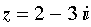$z=2-3i$$\text{Insert Text Here}$$x+2$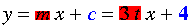$y=\colorbox[rgb]{1,0,0}{m}x+{c}=3tx+{\mathbf{4}}$$\left(\frac{x+2}{3}\right)$$\left(3,5,4\right)$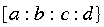$\left[a:b:c:d\right}$$〈p|a|\mathrm{p`}〉$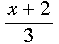$\frac{x+2}{3}$$\left(\genfrac{}{}{0}{}{2}{3}\right)$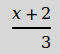$\frac{x+2}{\hfill 3}$$x+2}{3}$$\sqrt{x+2}$$\sqrt{x+2}$${x}_{i}$${e}^{x+2}$${x}_{2}^{i}$${{x}_{i}}^{2}$${\int }_{0}^{1}xdx$$\underset{⏟}{x}+\stackrel{^}{y}$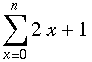$\sum _{x=0}^{n}2x+1$$G_{i}^{j}{}_{k}{}^{l}{}_{m}{}^{n}$$G_{i}{}_{k}{}^{l}{}^{n}$${}^{a}{}_{b}{}^{c}G_{i}{}_{k}{}^{l}$$\left(\begin{array}{ccc}1& 5& 3\\ 8& 2& 6\\ 7& 9& 0\end{array}\right)$$\left(\begin{array}{c}1\\ 8\\ 7\end{array}\right)$